# RD Sharma Solutions for Class 10 Chapter 1 Real Numbers Exercise 1.6

In earlier class, we would have learnt about the decimal expansion of a rational number that is either terminating or non-terminating repeating without the actual knowledge of when it’s terminating or non-terminating. In this exercise, the problems focus on making students understand exactly when the decimal expansion of a rational number is terminating and when it is non-terminating repeating. The RD Sharma Solutions Class 10 is the best resource available to learn the correct methods to solve such problems and secure high marks in the exams. Students can also get the RD Sharma Solutions for Class 10 Maths Chapter 1 Real Numbers Exercise 1.6 PDF, which is available below.

## RD Sharma Solutions for Class 10 Chapter 1 Real Numbers Exercise 1.6 Download PDF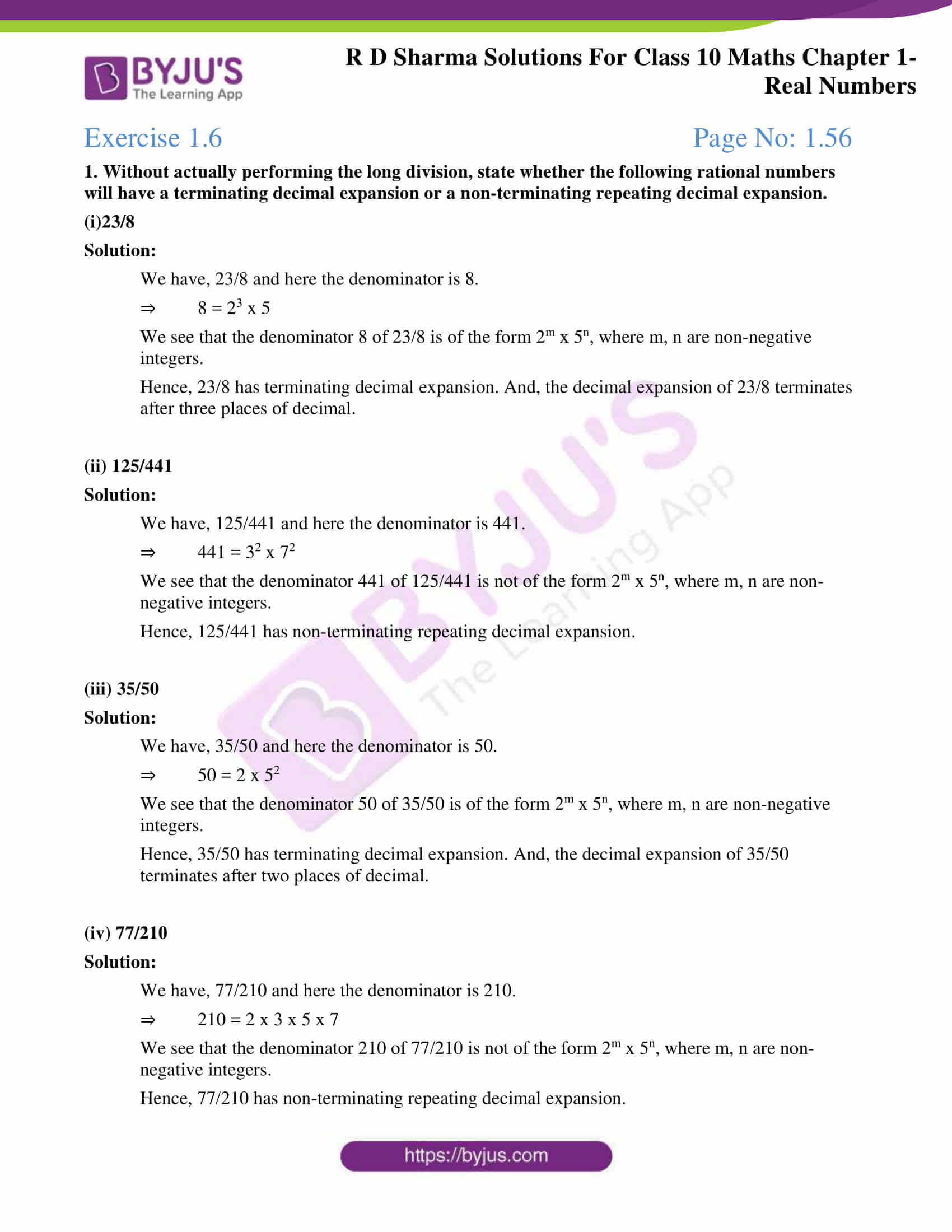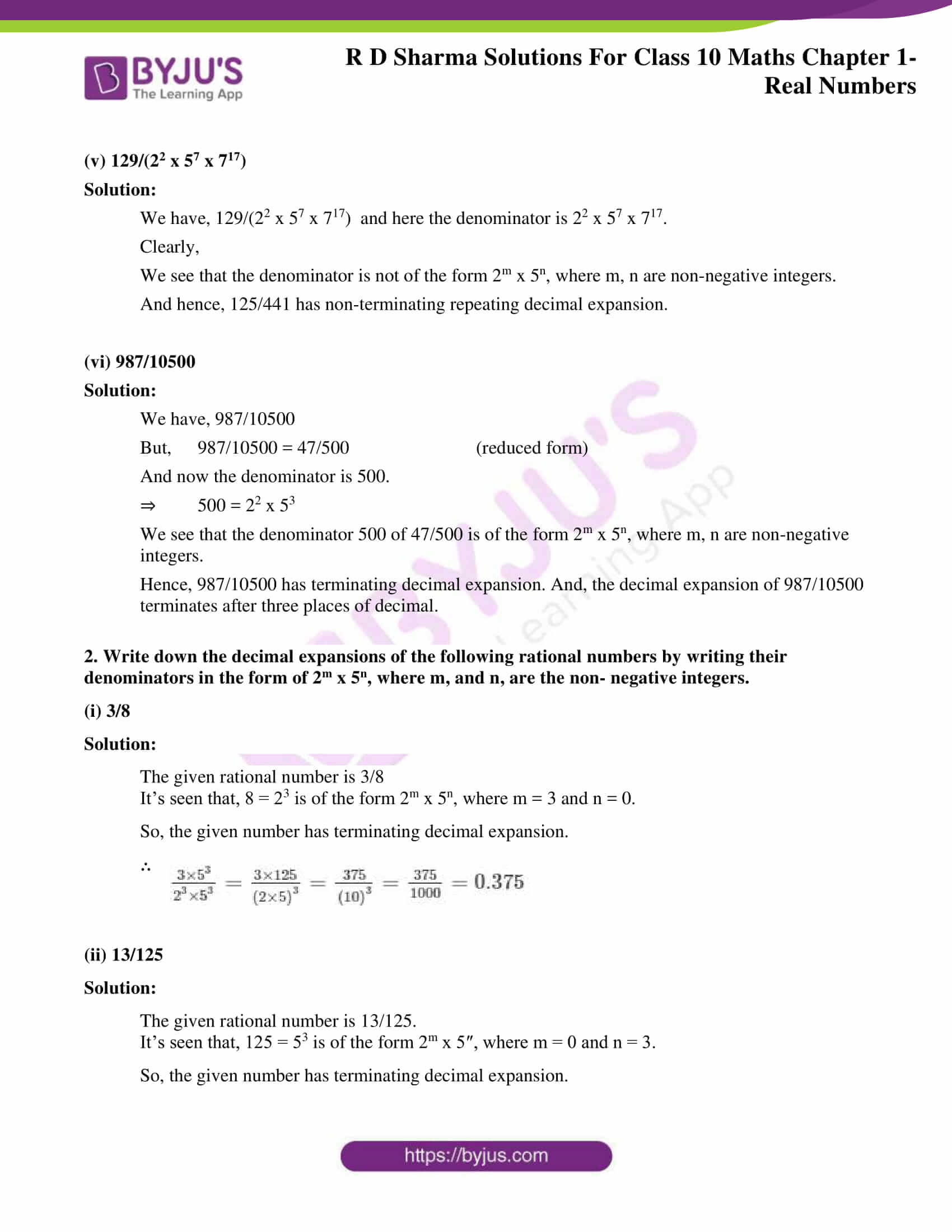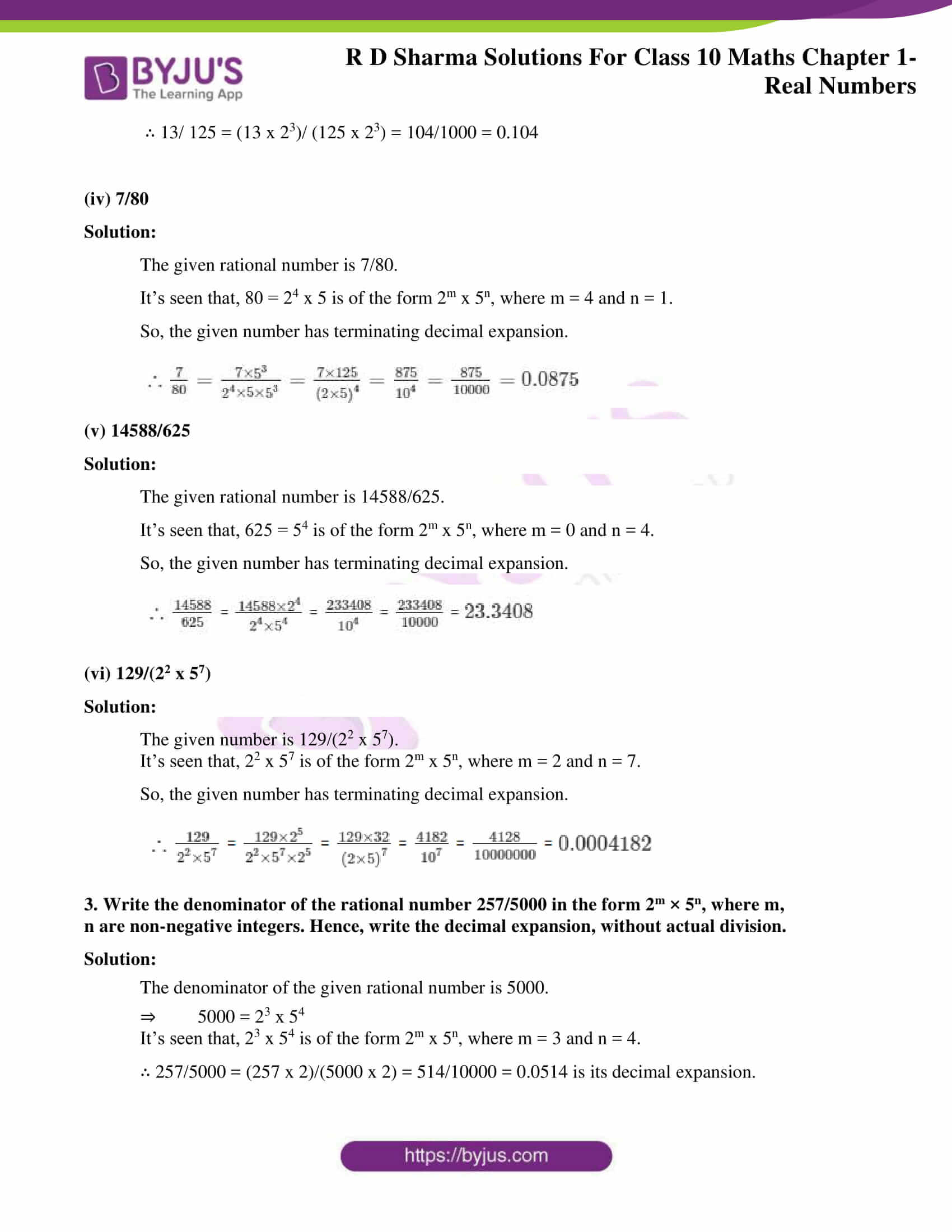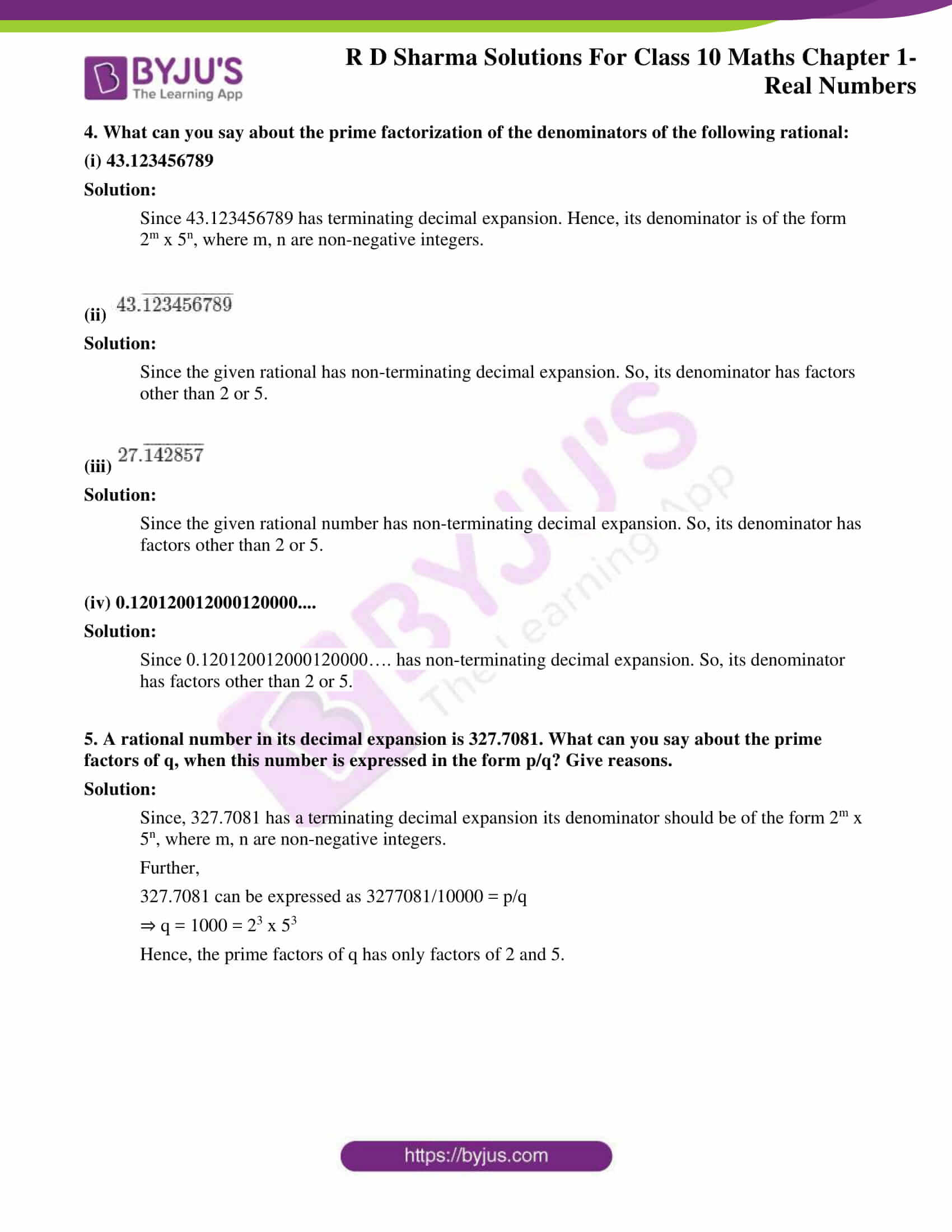### Access RD Sharma Solutions for Class 10 Chapter 1 Real Numbers Exercise 1.6

1. Without actually performing the long division, state whether the following rational numbers will have a terminating decimal expansion or a non-terminating repeating decimal expansion.

(i)23/8

Solution:

We have, 23/8 and here the denominator is 8.

⇒ 8 = 23 x 5

We see that the denominator 8 of 23/8 is of the form 2m x 5n, where m, n are non-negative integers.

Hence, 23/8 has terminating decimal expansion. And, the decimal expansion of 23/8 terminates after three places of decimal.

(ii) 125/441

Solution:

We have, 125/441 and here the denominator is 441.

⇒ 441 = 32 x 72

We see that the denominator 441 of 125/441 is not of the form 2m x 5n, where m, n are non-negative integers.

Hence, 125/441 has non-terminating repeating decimal expansion.

(iii) 35/50

Solution:

We have, 35/50 and here the denominator is 50.

⇒ 50 = 2 x 52

We see that the denominator 50 of 35/50 is of the form 2m x 5n, where m, n are non-negative integers.

Hence, 35/50 has terminating decimal expansion. And, the decimal expansion of 35/50 terminates after two places of decimal.

(iv) 77/210

Solution:

We have, 77/210 and here the denominator is 210.

⇒ 210 = 2 x 3 x 5 x 7

We see that the denominator 210 of 77/210 is not of the form 2m x 5n, where m, n are non-negative integers.

Hence, 77/210 has non-terminating repeating decimal expansion.

(v) 129/(22 x 57 x 717)

Solution:

We have, 129/(22 x 57 x 717) and here the denominator is 22 x 57 x 717.

Clearly,

We see that the denominator is not of the form 2m x 5n, where m, n are non-negative integers.

And hence, 125/441 has non-terminating repeating decimal expansion.

(vi) 987/10500

Solution:

We have, 987/10500

But, 987/10500 = 47/500 (reduced form)

And now the denominator is 500.

⇒ 500 = 22 x 53

We see that the denominator 500 of 47/500 is of the form 2m x 5n, where m, n are non-negative integers.

Hence, 987/10500 has terminating decimal expansion. And, the decimal expansion of 987/10500 terminates after three places of decimal.

2. Write down the decimal expansions of the following rational numbers by writing their denominators in the form of 2m x 5n, where m, and n, are the non- negative integers.

(i) 3/8

Solution:

The given rational number is 3/8

It’s seen that, 8 = 23 is of the form 2m x 5n, where m = 3 and n = 0.

So, the given number has terminating decimal expansion.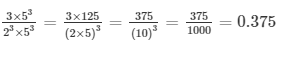(ii) 13/125

Solution:

The given rational number is 13/125.

It’s seen that, 125 = 53 is of the form 2m x 5″, where m = 0 and n = 3.

So, the given number has terminating decimal expansion.

∴ 13/ 125 = (13 x 23)/ (125 x 23) = 104/1000 = 0.104

(iv) 7/80

Solution:

The given rational number is 7/80.

It’s seen that, 80 = 24 x 5 is of the form 2m x 5n, where m = 4 and n = 1.

So, the given number has terminating decimal expansion.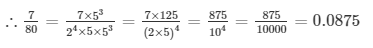(v) 14588/625

Solution:

The given rational number is 14588/625.

It’s seen that, 625 = 54 is of the form 2m x 5n, where m = 0 and n = 4.

So, the given number has terminating decimal expansion.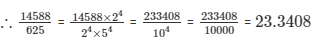(vi) 129/(22 x 57)

Solution:

The given number is 129/(22 x 57).

It’s seen that, 22 x 57 is of the form 2m x 5n, where m = 2 and n = 7.

So, the given number has terminating decimal expansion.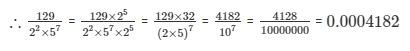3. Write the denominator of the rational number 257/5000 in the form 2m × 5n, where m, n are non-negative integers. Hence, write the decimal expansion, without actual division.

Solution:

The denominator of the given rational number is 5000.

⇒ 5000 = 23 x 54

It’s seen that, 23 x 54 is of the form 2m x 5n, where m = 3 and n = 4.

∴ 257/5000 = (257 x 2)/(5000 x 2) = 514/10000 = 0.0514 is its decimal expansion.

4. What can you say about the prime factorization of the denominators of the following rational:

(i) 43.123456789

Solution:

Since 43.123456789 has terminating decimal expansion. Hence, its denominator is of the form 2m x 5n, where m, n are non-negative integers.

(ii)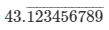Solution:

Since the given rational has non-terminating decimal expansion. So, its denominator has factors other than 2 or 5.

(iii)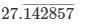Solution:

Since the given rational number has non-terminating decimal expansion. So, its denominator has factors other than 2 or 5.

(iv) 0.120120012000120000….

Solution:

Since 0.120120012000120000…. has non-terminating decimal expansion. So, its denominator has factors other than 2 or 5.

5. A rational number in its decimal expansion is 327.7081. What can you say about the prime factors of q, when this number is expressed in the form p/q? Give reasons.

Solution:

Since, 327.7081 has a terminating decimal expansion its denominator should be of the form 2m x 5n, where m, n are non-negative integers.

Further,

327.7081 can be expressed as 3277081/10000 = p/q

⇒ q = 1000 = 23 x 53

Hence, the prime factors of q has only factors of 2 and 5.